Courses

# OP–amp Nat Level – 1

## 10 Questions MCQ Test Topic wise Tests for IIT JAM Physics | OP–amp Nat Level – 1

Description
This mock test of OP–amp Nat Level – 1 for Physics helps you for every Physics entrance exam. This contains 10 Multiple Choice Questions for Physics OP–amp Nat Level – 1 (mcq) to study with solutions a complete question bank. The solved questions answers in this OP–amp Nat Level – 1 quiz give you a good mix of easy questions and tough questions. Physics students definitely take this OP–amp Nat Level – 1 exercise for a better result in the exam. You can find other OP–amp Nat Level – 1 extra questions, long questions & short questions for Physics on EduRev as well by searching above.
*Answer can only contain numeric values
QUESTION: 1

### A bistable multi vibrator with a saturation voltage 15Vis shown in the diagram. The magnitude of the positive and negative thresholds at the inverting terminal for which the multi vibrator will switch to the other state are in volts (up to 2 decimal places).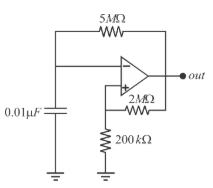Solution:

The threshold voltage is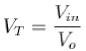where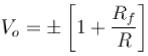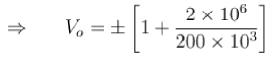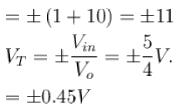*Answer can only contain numeric values
QUESTION: 2

### A certain Op-Amp has bias currents of 50μA and 49.3μA. The input offset current is (in nA).

Solution:

The input offset current is the different of input bias currents i.e.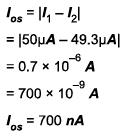*Answer can only contain numeric values
QUESTION: 3

### A certain non-inverting amplifier has an Ri equa l to 1.0kΩ and Rf . equal to 1.0kΩ. The close loop gain is :

Solution: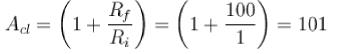*Answer can only contain numeric values
QUESTION: 4

The Common Mode Rejection Ratio (CMRR) of a differential amplifier using an operational amplifier is 100 dB. The output voltage for a differential input of is 2V. The common mode gain is (in dB)

Solution: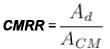Where Ad = differential voltage gain
= large signal voltage gains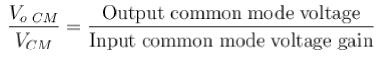= Common mode voltage gain
The CMRR is generally expressed is dB and denoted by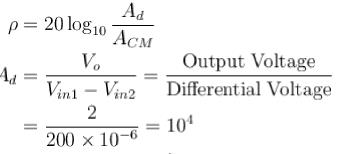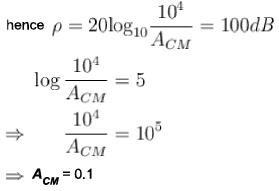*Answer can only contain numeric values
QUESTION: 5

If a certain Op-Amp has a mid range open-loop gain of 200000 and a unity-gain frequency of 5MHz, the gain bandwidth product in Hz is :

Solution:

gain bandwidth product = frequency at which gain is unity
= 5 MHz
= 5000000 Hz

*Answer can only contain numeric values
QUESTION: 6

A certain Op-Amp has three terminal amplifier stages with the following gains
Stage I : Av1 = 40 dB
Stage I : Av2 = 32 dB
Stage I : Av3 = 20 dB
The open-loop mid range gain in decibels?

Solution: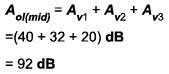*Answer can only contain numeric values
QUESTION: 7

The output V0 of the ideal Op-Amp circuit shown in the figure is (in Volts)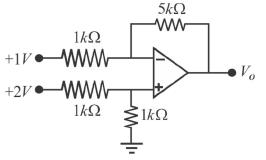Solution: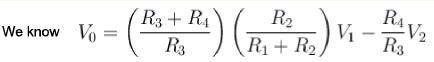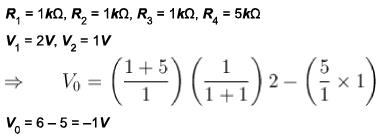*Answer can only contain numeric values
QUESTION: 8

Given the Op-Amp configuration is given figure below, the value of the Rf required to produce a closed - loop voltage gain of-100. (in kΩ).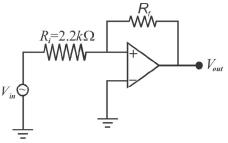Solution: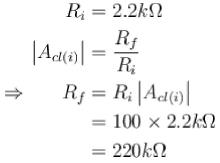*Answer can only contain numeric values
QUESTION: 9

The gain of the amplifier shown in figure. The open loop voltage gain of the Op-Amp is 1,00,000.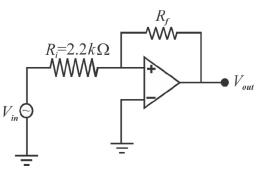This is a non-inverting Op-Amp configuration. Therefore, the closed loop voltage gain is

Solution:

This is a non-inverting Op-Amp configuration. Therefore, the closed loop voltage gain is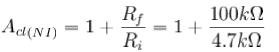= 22.3

*Answer can only contain numeric values
QUESTION: 10

The output voltage of a certain Op-Amp appears as shown in figure in response to a step input. What is the slew rate (in V/μs)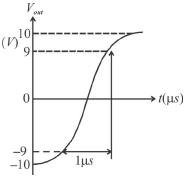Solution:

The output goes from the lower to the upper limit. Since, this response is not ideal, the limit are taken at the 90% points, as indicated. So the upper limit is + 9Vand the lower limit is - 9V. The slew rate is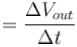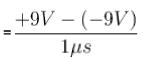= 18 V/μs